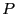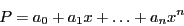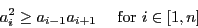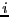Next: Implementation Up: Du Gua-Huat-Euler theorem Previous: Du Gua-Huat-Euler theorem   Contents

Mathematical background

Letthe polynomial:If all the roots ofare real then :Conversely if this relation does not hold for some(for example if a coefficient is 0 while its neighbor have same sign), then there are complex roots.

Jean-Pierre Merlet 2012-12-20